# GSEB Solutions Class 11 Maths Chapter 9 Sequences and Series Miscellaneous Exercise

Gujarat Board GSEB Textbook Solutions Class 11 Maths Chapter 9 Sequences and Series Miscellaneous Exercise Textbook Questions and Answers.

## Gujarat Board Textbook Solutions Class 11 Maths Chapter 9 Sequences and Series Miscellaneous Exercise

Question 1.
Show that the sum of (m + n)th and (m – n)th terms of an A.P. is equal to the twice of the mth term?
Solution:
Let a be the first term and d be the common difference of an A.P.
Now we want to prove that
Tm+n + Tm-n = 2Tm
L.H.S. = Tm+n + Tm-n
= [a + (m + n – 1)d] + [a + (m – n – 1)d]
= 2a + (m + n – 1 + m – n – 1 )d
= 2a + (2m – 2)d = 2[a + (m – 1)d]
= 2Tm = R.H.S.Question 2.
If the sum of three numbers in A.P. is 24 and their product is 440, find the numbers?
Solution:
Let the three numbers in A.P. be a – d, a, a + d
Their sum = (a – d) + a + (a + d) = 24
⇒ 3a = 24
⇒ a = 8.
Their product = (a – d)(a)(a + d) = 440.
⇒ a(a2 – d2) = 440
⇒ 8(64 – d) = 440
⇒ 64 – d2 = $$\frac{440}{8}$$ = 55
⇒ d2 = 64 – 55 = 9 ⇒ d = ± 3.
Hence, the numbers are 8 – 3, 8 + 3 or 8 + 3, 8 – 3.
i.e., 5, 8, 11 or 11, 8, 5.Question 3.
Let the sum of first n, 2n and 3n terms of an A.P. be S1? S2 and S3 respectively. Show that S3 = 3(S2 – S1).
Solution:
Let a be the first term and d be the common difference of A.P.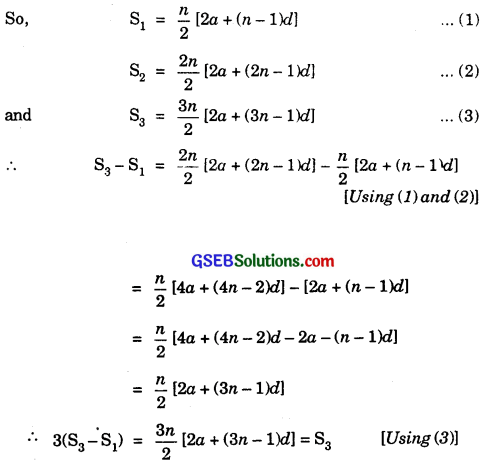Hence, S3 = 3(S2 – S1).

Question 4.
Find the sum of all numbers between 200 and 400, which are divisible by 7?
Solution:
The numbers between 200 and 400, which are divisible by 7, are 203, 210, 217, 224, …, 399.
Here, a = 203, d = 7, l = Tn = 399.
Since Tn = a + (n – 1)d. So,
399 = 203 + (n – 1)7
⇒ 399 – 203 = (n – 1)7
⇒ 196 = (n – 1)7
i.e., 29 numbers lie between 200 and 400, which are divisible by 7.
Since Sn = $$\frac{n}{2}$$(a + l), therefore
Sn = $$\frac{29}{2}$$(203 + 399) = $$\frac{29}{2}$$ × 602
= 29 × 301 = 8729.Question 5.
Find the sum of integers from 1 to 100 that are divisible by 2 or 5?
Solution:
Sum of the numbers from 1 to 100 that are divisible by 2 = 2 + 4 + 6 + … + 100
= $$\frac{50}{2}$$ [2 × 2 + (50 – 1).2]
= 25[4 + 49 × 2] = 25 × (4 + 98)
= 25 × 102 = 2550.
Sum of the numbers which are divisible by 5 but not by 2
= 5 + 15 + 25 + … + 95
Now, 95 = 5+ (n – 1)10
But n – 1 = 9. So, n = 10
Now, 5 + 15 + 25 + … + 95 = $$\frac{10}{2}$$ [2 × 5 + (10 – 1).10]
= 5(10 + 90) = 500.
∴ Sum of the integers from 1 to 100 that are divisible by 2 or 5
= 2550 + 500
= 3050.Question 6.
Find the sum of all two digit numbers which when divided by 4, yields 1 as remainder?
Solution:
The sum of two digit numbers which when divided by 4 yield 1 as remainder is
13 + 17 + 21 + … + 97.
Let the sum be denoted by S and let 97 be the nth term.
∴ 97 = a + (n – 1)d = 13 + (n – 1)4 [∵ a = 13, d = 4]
∴ 97 – 13 = 4(n – 1)
or 84 = 4(n – 1) ]
⇒ n – 1 = 21
⇒ n = 22.
∴ The sum of the series
S = 13 + 17 + 21 + … + 97
[Put a = 13, d = 4 and n = 22 in $$\frac{n}{2}$$ [2a + (n – 1 )d]
= $$\frac{22}{2}$$ [2 × 13 + (22 – 1).4]
= 11(26 + 21 × 4)
= 11(26 + 84) = 11 × 110 = 1210.Question 7.
If f is a function satisfying f(x + y) = f(x)f(y) for all x, y ∈ N such that f(1) = 3 and $$\sum_{1}^{n}$$ f(x) = 120, find the value of n?
Solution:
f(1) = 3, f(x + y) = f(x)f(y),
∴ f(2) = f(1 + 1) = f(1).f(1)
= 3.3 = 9,
f(3) = f(1 + 2) = f(1)f(2) = 3.9 = 27
and f(4) = f(1 + 3) = f(1).f(3) = 3.27 = 81.
Thus, we have:
$$\sum_{1}^{n}$$f(x) = f(1) + f(2) + f(3) + …………….. + f(n) = 120.
or 3 + 9 + 27 + … to n terms = 120.
3n = 80 + 1 = 81 = 34
∴ n = 4.Question 8.
The sum of some terms of a G.P. is 315, whose first term and the common ratio are 5 and 2, respectively. Find the last term and the number of terms?
Solution:
Let Sn = 315, a = 5, r = 2.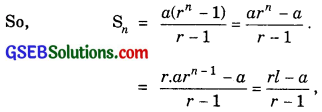where l = last term = Tn.
∴ 315 = $$\frac{2l – 5}{2 – 1}$$ ⇒ 315 = 2l – 5.
⇒ 2l = 315 + 5 = 320 ⇒ l = $$\frac{320}{2}$$ = 160.
Now, l = Tn = arn-1
⇒ 160 = 5(2)n-1 ⇒ 2n-1 = $$\frac{160}{5}$$ = 32.
⇒ 2n-1 = (2)5 ⇒ n – 1 = 5, i.e; n = 6.

Question 9.
The first term of a G.P. is 1. The sum of the third and fifth terms is 90. Find the common ratio of G.P?
Solution:
Here, 1 is the first term. Let r be the common ratio of the G.P.
Given: T3 + T5 = 90
⇒ 1.r3-1 + 1.r5-1 = 90
⇒ r2 + r4 = 90
r4 + r2 – 90 = 0
Put r2 = y. Then, (1) becomes
y2 + y – 90 = 0
⇒ (y + 10)(y – 9) = 0
⇒ y = – 10 or y = 9
⇒ r2 = – 10 (rejected) or r2 = 9.
⇒ r = ± 3.
Hence, the common ratio = ± 3.Question 10.
The sum of three numbers in G.P. is 56. If we subtract 1, 7, 21 from these numbers in that order, we obtain an arithmetic progression. Find the numbers?
Solution:
Let the numbers in G.P. be a, ar, ar2.
Their sum = a + ar + ar2 = 56 ………………… (1)
On subtracting 1, 7, 21 from a, ar, ar2 respectively, the new numbers a – 1, ar – 7 and ar2 – 21 are given to be in A.P.
∴ 2(ar – 7) = (a – 1) + (ar2 – 21)
⇒ 2ar – 14 = a – 1 + ar2 – 21
⇒ ar2 – 2ar + a = 8 ………………. (2)
(1) – (2) gives:
⇒ ar = $$\frac{48}{3}$$ = 16.
⇒ a = $$\frac{16}{r}$$ …………………. (3)
Put a = $$\frac{16}{r}$$ in (1), we get
$$\frac{16}{r}$$ + 16 + 16r = 56.
⇒ 16 + 16r + 16r2 = 56r ⇒ 6r2 – 40r + 16 = 0.
⇒ 2r2 – 5r + 2 = 0 ⇒ 2r2 – 4r – r + 2 = 0.
⇒ 2r(r – 2) – 1(r – 2) = 0 ⇒ (r – 2)(2r – 1) = 0.
∴ r = 2 or r = $$\frac{1}{2}$$.
When r = 2, then a = $$\frac{16}{8}$$ = 8.
Then, the numbers are 8, 16, 32.
When r = $$\frac{1}{2}$$, then a = $$\frac{16}{1/2}$$ = 32.
Then, the numbers are 32, 16, 8.Question 11.
A G.P. consists of an even number of terms. If the sum of all the terms is 5 times the sum of the terms occupying odd places, then find its common ratio?
Solution:
Let the G.P. be
S = a + ar + ar2 + … to 2n terms
= $$\frac{a\left(r^{2 n}-1\right)}{r-1}$$
The series formed by taking terms occupying odd places is
S1 = a + ar2 + ar4 + … to n termsNow, S = 5S1⇒ 1 = $$\frac{5}{r+1}$$
[Cancelling the common factor $$\frac{a\left(r^{2 n}-1\right)}{r-1}$$]
or r + 1 = 5.
∴ r = 4.

Question 12.
The sum of first four terms of an A.P. is 56. The sum of last four terms is 112. If its first term is 11, then find the number of terms?
Solution:
The sum of first four terms of the A.P.
= 11 + (11 + d) + (11 + 2d) + (11 + 3d)
= $$\frac{4}{2}$$ [11 × 2 + (4 – 1)d]
[Putting a = 11, n = 4 in $$\frac{n}{2}$$[2a + (n – 1)d]
= 2[22 + 3d] = 44 + 6d = 56 …………….. (1)
6d = 56 – 4 = r
∴ d = 2.
The last four terms are Tn-3, Tn-2, Tn-1, Tn.
Tn-3 = a + (n – 4)d
= 11 + (n – 4)d.
∴ Sum of last four terms
= $$\frac{4}{2}$$[2(11) + (n – 4)d] + (4 – 1)d].
= 2[22 + 2nd – 8d + 3d]
= 2[22 + 2nd – 5d] = 112
or 22 + 2nd – 5d = 56 ………………. (2)
Putting d = 2 in (2), we get
22 + 4n – 10 = 56.
or 4x = 56 – 12 = 44
n = 11.Question 13.
If $$\frac{a+bx}{a-bx}$$ = $$\frac{b+cx}{b-cx}$$ = $$\frac{c+dx}{c-dx}$$, then show that a, b, c, d are in G.P.
Solution:
We have:
$$\frac{a+bx}{a-bx}$$ = $$\frac{b+cx}{b-cx}$$ = $$\frac{c+dx}{c-dx}$$,
Applying componendo and dividendo, we get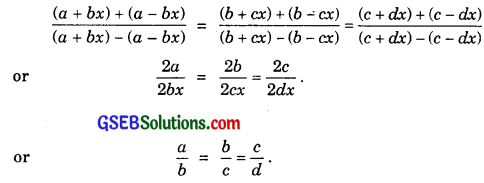Hence a, b, c, d are in G.P.

Question 14.
Let S be the sum, P the product and R the sum of reciprocals of first n terms in a G.P. Prove that P2R2 = Sn.
Solution:
Let the sum of first n terms be a + ar + ar2 + where r < 1 (say).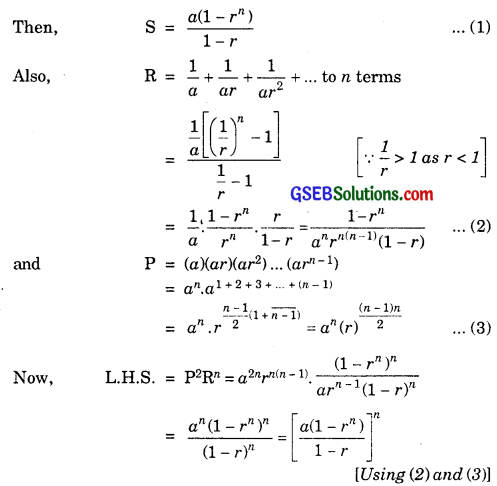= Sn = R.H.S. [Using (1)]Question 15.
The pth, qrth and rth terms of an A.P. are a, b and c respectively show that
(q – r)a + (r – p)b + (p – q)c = 0.
Solution:
Let A be the first term and D be the common difference of the A.P.
∴ Tp = A + (p – 1)D = a ……………… (1)
Tq = A + (q – 1)D = b ……………….. (2)
Tr = A + (r – 1)D = c …………………. (3)
Multiply (1) by q – r, (2) by r – p and (3) by p – q and add.
[A(q – r)+ (p – 1)(q – r)D] + [A(r – p) + (q – 1)(r – p)D] + [A(p – q) + (r – 1)(p – q)D] = a(q – r) + b(r – b) + c(p – q)
∴ a(q – r) + b(r – b) + c (p – q)
= A[(q – r) + (r – p) + (p – q)] + D(p – 1)(q – r) +(q – 1)(r – p) + (r – 1)[(p – q)]
= D[p(q – r) + q(r – p) + r(p – q) – (q – r + r – p + p – q)
= D × 0 = 0.

Question 16.
If a($$\frac{1}{b}$$ + $$\frac{1}{c}$$), b($$\frac{1}{c}$$ + $$\frac{1}{a}$$), c($$\frac{1}{a}$$ + $$\frac{1}{b}$$), are in A.P., prove that a, b, c are in A.P.
Solution:
We have:
a($$\frac{1}{b}$$ + $$\frac{1}{c}$$), b($$\frac{1}{c}$$ + $$\frac{1}{a}$$), c($$\frac{1}{a}$$ + $$\frac{1}{b}$$) are in A.P.
Adding 1 to each term, we get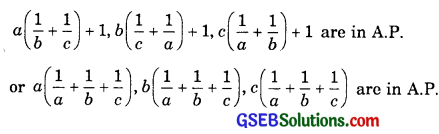Dividing by $$\frac{1}{a}$$ + $$\frac{1}{b}$$ + $$\frac{1}{c}$$, we get
a, b, c are in A.P.Question 17.
If a, b, c, d are in G.P. prove that
(an + bn), (bn + cn), (cn + dn) are in G.P.
Solution:
Since a, b, c, d are in G.P., therefore
$$\frac{b}{a}$$ = $$\frac{c}{b}$$ = $$\frac{d}{c}$$ = r(say)
⇒ b = ar, c = br = (ar)r = ar2,
and d = cr = (ar2)r = ar3.
Now an + bn, bn + cn, cn + dn are in G.P. if
(bn + cn)2 = (an + bn)(cn + dn).
Now, L.H.S. = (bn + cn)2 = [(ar)n + (ar2)n]2
= [anrn(1 + rn)] = a2nr2n(1 + rn)2
Also, R.H.S. = (an + bn)(cn + dn)
= (an + anrn)(anr2n + anr3n)
= an(1 + rn).anr2n(1 + r)n
= a2nr2n(1 + rn)2
∴ L.H.S. = R.H.S.

Question 18.
If a and b are the roots of x2 – 3x + p = 0 and c and d are the roots of x2 – 12x + q = 0, where a, b, c, d form a G.P., prove that (q + p) : (q – 5) = 17 : 15.
Solution:
a and b the roots of x2 – 3x + p = 0.
∴ a + b = 3, ab = p ……………. (1)
[∵ Sum of the roots = – $$\frac{b}{a}$$, Product of roots = $$\frac{c}{a}$$]
In equation ax2 + bx + c = 0 , c and d are the roots of
x2 – 12x + q = 0.
∴ c + d = 12 and cd = q …………….. (2)
Also a, b, c and d are in G.P. Let r be its common ratio.
∴ b = cr, c = ar2, d = ar3 ………………. (3)
Now, a + b = a + ar = 3
and c + d = ar2 + ar3 = 12.
Dividing, we getPutting values of b, c and d from (3), we get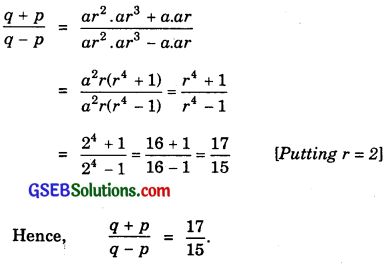Question 19.
Let the ratio of the A.M. and G.M. of two positive numbers a and b be m : n. Show that
a : b = (m + $$\sqrt{m^{2}-n^{2}}$$) : (m – $$\sqrt{m^{2}-n^{2}}$$).
Solution:
As per question,Applying componendo and dividendo, we have: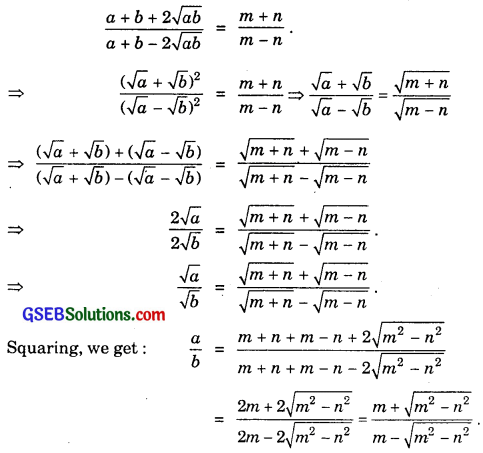Question 20.
If a, b and c are in A.P; b, c and d are in G.P. and $$\frac{1}{c}$$, $$\frac{1}{d}$$ and $$\frac{1}{e}$$ are in A.P., prove that a, c and e are in G.P.
Solution:
a, b and c are in A.P. ⇒ b = $$\frac{a+c}{2}$$.
b, c and d are in G.P. ⇒ c2 = bd …………….. (2)
$$\frac{1}{c}$$, $$\frac{1}{d}$$ and $$\frac{1}{e}$$ are in A.P.
∴ $$\frac{2}{d}$$ = $$\frac{1}{c}$$ + $$\frac{1}{e}$$
⇒ d = $$\frac{2ce}{c+e}$$ …………………. (3)
Putting the values of b and d, from (1) and (3), in (2), we get
c2 = $$\frac{a+c}{2}$$.$$\frac{2ce}{c+e}$$ = $$\frac{ce(a+c)}{c+e}$$
⇒ c(c + e) = e(a + c)
or c2 + ce = ae + ce
⇒ c2 = ae
Hence a, c and e are in G.P.Question 21.
Find the sum of the following series upto n terms:
(i) 5 + 55 + 555 + …
(ii) 0.6 + 0.66 + 0.666 + …
Solution:
(i) Let Sn = 5 + 55 + 555 + … to n terms
= 5[1 + 11 + 111 + … to n terms](ii) Let Sn = 0.6 + 0.66 +0.666 + ………….. to n terms
= 6[0.1 + 0.11 + 0.111 + …………. to n terms]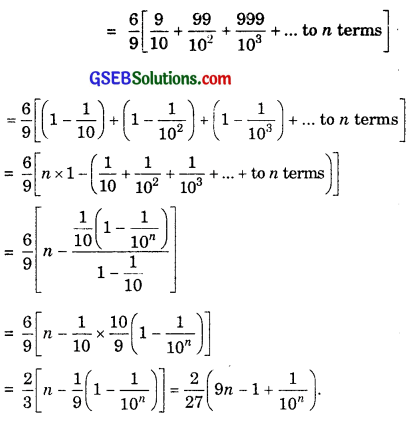Question 22.
Find the 20th term of the series
2 × 4 + 4 × 6 + 6 × 8 + … to n terms.
Solution:
First factor of the terms are 2, 4, 6, ……
∴ First factor of nth term = 2n. …………….. (1)
∴ Second factor of the terms are 4, 6, 8, …
∴ Second factor of nth term
= 4 + [n – 1].2 = 2n + 2
= 2(n + 1) …………… (2)
∴ nth term of the given series
= 2n × 2(n + 1) [From (1)and (2)]
= 4n(n + 1)
∴ Putting n = 20, we get
20th term of the given series
= 4 × 20 × 21
= 80 × 21
= 1680.Question 23.
Find the sum of the first n terms of the series 3 + 7 + 13 + 21 + 31 + ……
Solution:
Let Sn be the required sum and Tn be the nth term of the series.
Then, Sn = 3 + 7 + 13 + 21 + 31 + … + Tn …………… (1)
Also, Sn = 3 + 7 + 13 + … + Tn-1 + Tn ………………. (2)
(1) – (2) gives:
0 = 3 + 4 + 6 + 8 + 10 + … to n terms – Tn
⇒ Tn = 3 + (4 + 6 + 8 + 10 + … to n – 1 terms)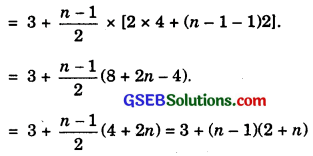= 3 + n2 + n – 2 = n2 + n + 1.Question 24.
If S1, S2 and S3 are the sums of first n natural numbers, their squares and their cubes respectively, show that 9S22 = S3(1 + 8S1).
Solution:
S1 is sum of the first n natural numbers.
∴ S1 = $$\frac{n(n+1)}{2}$$.
S2 is the sum of the squares first n natural numbers.
∴ S2 = $$\frac{n(n+1)(2n+1)}{6}$$.
S3 is the sum of the cubes of first n natural numbers.
∴ S3 = {$$\frac{n(n+1)}{2}$$}2.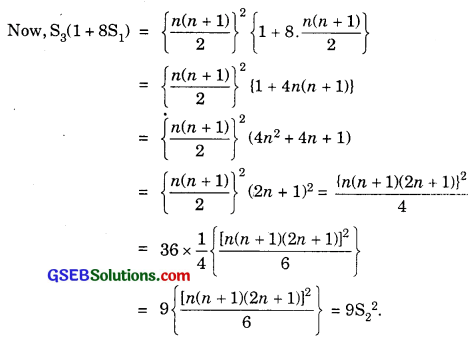Question 25.
Find the sum of the following series upto n terms.
$$\frac{1^{3}}{1}+\frac{1^{3}+2^{3}}{1+3}+\frac{1^{3}+2^{3}+3^{3}}{1+3+5}$$ + ………………
Solution: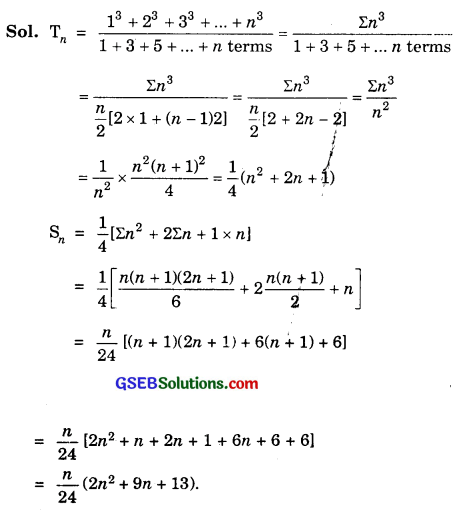Question 26.
Show that $$\frac{1 \times 2^{2}+2 \times 3^{2}+\ldots+n \times(n+1)^{2}}{1^{2} \times 2+2^{2} \times 3+\ldots+3^{2} \times(n-1)}$$ = $$\frac{3n+5}{3n+1}$$.
Solution:
Let numerator S1 = 1 × 22 + 2 × 32 + …………. + n × (n + 1)2.
Tn = n(n + 1)2 = n(n2 + 2n + 1)
= n3 + 2n2 + n.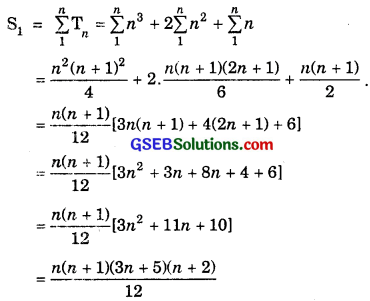The denominator is denoted by S2
S2 = 12 × 2 + 22 × 3 + ………….. + n2 × (n + 1).
Its nth term = n2(n + 1) = n3 + n2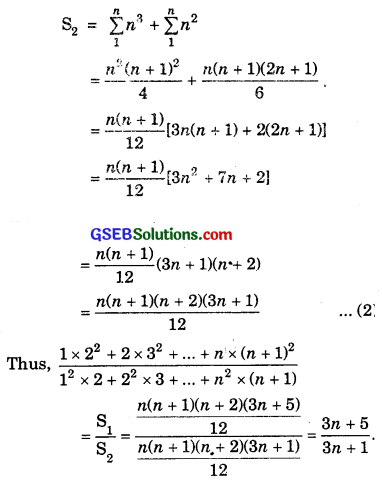[From (1) and (2), values of S1 and S2 are substituted]Question 27.
A farmer buys a used tractor for ₹ 12000. He pays ₹ 1000 cash and agrees to pay the balance in annual instalments of ₹ 500 plus 12% interest on the unpaid amount. How much will the tractor cost him?
Solution:
Total cost of the tractor = ₹ 12000.
Paid cash = ₹ 6000.
Balance = ₹ 6000.
Number of instalments @ ₹ 500 each = 12.
Interest on first instalment = ₹ ($$\frac{6000×12×1}{100}$$)
= ₹ 720.
∴ First instalment = ₹ (500 + 720) = ₹ 1220.
Interest on second instalment = ₹ ($$\frac{5500×12×1}{100}$$)
= ₹ 660.
∴ Second instalment = ₹ (500 + 660) = ₹ 1160.
Interest on third instalment = ₹ ($$\frac{5500×12×1}{100}$$)
= ₹ 600.
∴ Third instalment = ₹ (500 + 600)
= ₹ 1100 and so on.
∴ Total amount paid in instalments
= ₹ (1220 + 1160 + 1100 + … to 12 terms)
S = $$\frac{12}{2}$$[2 × 1220 + (12 – 1) (- 60)] [∵ it is an A.P.]
= 6[2440 – 11 × 60]
= 6(2440 – 660) = 6 × 1780 = 10680
= ₹ 10680.
∴ Amount paid by farmer = ₹ (6000 + 10680)
= ₹ 16680.Question 28.
Shamshad Ali buys a scooter for ₹ 22000. He pays ₹ 4000 cash and agrees to pay balance in annual instalments of ₹ 1000 plus 18% interest on the unpaid amount. How much will the scooter cost him?
Solution:
Total cost of a scooter = ₹ 22000.
Paid cash = ₹ 4000.
Balance = ₹ 18000.
Number of instalments @ ₹ 1000 each = 18
Interest on first instalment = ₹ ($$\frac{1800×10×1}{100}$$)
= ₹ 1800.
∴ First instalment = ₹ (1000 + 1800) = ₹ 2800.
Interest on second instalment = ₹ ($$\frac{1700×10×1}{100}$$)
= ₹ 1700.
∴ Second instalment = ₹ (1000 + 1700) = ₹ 2700.
Interest on third instalment = ₹ ($$\frac{1600×10×1}{100}$$)
= ₹ 1600.
Third instalment = ₹ (1000 + 1600)
= ₹ 2600 and so on
∴ Total amount paid in instalments
= ₹ (2800 + 2700 + 2600 + ………….. to 18 terms)
a = 2800, d = – 100, n = 18
S = $$\frac{18}{2}$$ [2 × 2800 + (18 – 1)(- 100)]
= 9(5600 – 1700) = 9 × 3900
= 35100.
Scooter will cost him ₹ (4000 + 35100) = ₹ 39100.Question 29.
A person writes a letter to four of his friends. He asks each one of them to copy the letter and mail to four different persons with the instruction that they move the chain similarly. Assuming that the chain is not broken and that it costs 50 paise to mail one letter, find the amount spent on postage, when 8th set of letters is mailed.
Solution;
As per question, the number of letters posted will form a G.P.
4, 16, 64, 256, …
Here, a = 4, c.r. = r = 4.
Number of total letters mailed upto 8th set of letters∴ Postage spent @ 50 paise per letter
= ₹ ($$\frac{50}{100}$$ × 87380) = ₹ 43690.

Question 30.
A man deposited ₹ 10000 in a bank at the rate of 5% simple interest annually. Find the amount in 15th year, since he deposited the amount and also calculate the total amount after 20 years?
Solution:
Initial deposit = ₹ 10000.
Rate of interest = 5% p.a. S.I.
∴ Interest for one year = ₹ ($$\frac{10000×5×1}{100}$$ = ₹ 17000.
∴ Amount in 15th year since deposit
= ₹ (10000 + 14 × 500) = ₹ 17000.
Amount after 20 years = ₹ (10000 + 20 × 500)
= ₹ 20000.Question 31.
A manufacturer reckons that value of a machine, which costs him ₹ 15625, will depreciate each year by 20%. Find the estimated value at the end of 5 years?
Solution:
The value of the machine, being depreciated, after n years will be
= p(1 – $$\frac{r}{100}$$)n
where P is the initial value of the machine, r is the rate at which it is depreciated.
Here, P = 15625, r = 20%, n = 5.
Depreciated value of the machine after 5 years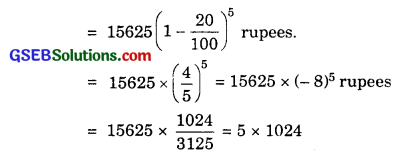= 5120 rupees.
Hence, the depreciated value of the machine after 5 years = ₹ 5120.Question 32.
150 workers were engaged to finish a job in a certain number of days. 4 workers dropped out on second day, 4 more workers dropped out on third day and so on. It took 8 more days to finish the work. Find the number of days in which the work was completed?
Solution:
Let 150 workers completed work in n days.
∴ 150 workers complete $$\frac{1}{n}$$ work in one day.
1 worker completes $$\frac{1}{150n}$$ work in one day.
∴ 150 workers complete $$\frac{150}{150n}$$ work in one day.
Since 4 workers are dropped on the second day, therefore
on second day’s work done by 146 workers = $$\frac{146}{150n}$$,
on second day’s work done by 142 workers = $$\frac{142}{150n}$$.
In this manner, the work was finished in (n + 8) days.
or $$\frac{1}{150n}$$ [50 + 146 + 142 + …………. to (n + 8) terms = 1.
∴ 150 + 146 + 142 + …………. to (n + 8) terms = 150n.
or $$\frac{n+8}{2}$$ [2 × 150 + (n + 8 – 1) terms] = 150n.
or (n + 8) [300 – 4(n + 7)] = 300n.
or (n + 8)(300 – 28 – 4n) = 300n.
2176 + 240n – 4n2 = 300n.
or 4n2 + 60n – 2176 = 0.
or n2 + 15n – 544 = 0.
or (n + 32)(n – 17) = 0.
∴ n = 17, n ≠ – 32.
∴ Work was completed in 17 + 8 = 25 days.